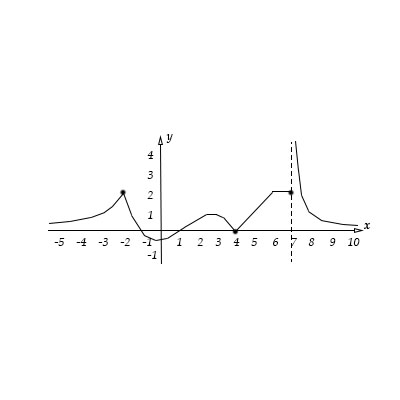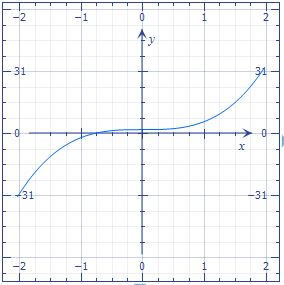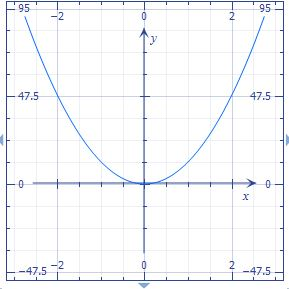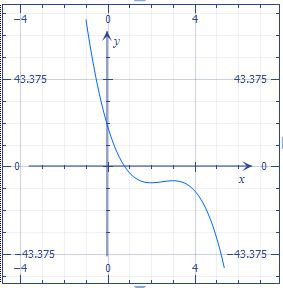# Given the graph of the function below, give a reasonable sketch of the derivative. a. Does...

## Question:

Given the graph of the function below, give a reasonable sketch of the derivative.

a. Does {eq}f(x)=x^4+2x+8 {/eq} have any inflection points? Where is this function concave up?

b. Graph {eq}f(x)=-2x^3+15x^2-36x+20. {/eq}## Concavity and Inflection Point:

With the second derivative of the function, we can find inflection points (points where the concavity of the function changes) in addition, we can know if the function is concave up or concave down

a. We have the function

{eq}f(x)=x^{4}+2x+8 \\ {/eq}

Differentiating the function

{eq}f'(x)=4\,{x}^{3}+2 \\ f''(x)=12\,{x}^{2} \\ {/eq}

Graph the function {eq}f'(x)=4\,{x}^{3}+2 \\ {/eq}Graph the function {eq}f''(x)=12\,{x}^{2} \\ {/eq}{eq}f''(x)=0 {/eq} The function has no real solution. Therefore, The function has no inflection point.

{eq}\begin{array}{r|D{.}{,}{5}} Interval & {-\infty<x< \infty } \\ \hline Test \space{} value & \ x=1 \\ Sign \space{} of \ f'' (x) & \ f'' (1)>0 \\ Conclusion & concave \space up \\ \end{array} \\ {/eq}

b. Graph the function {eq}f(x)=-2x^3+15x^2-36x+20. {/eq}